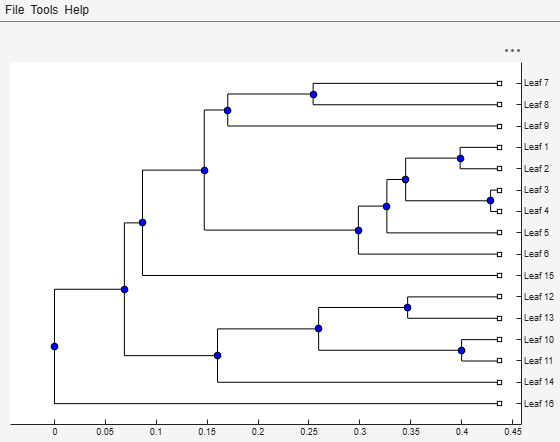# phytree

Create phytree object

## Syntax

```Tree = phytree(B) Tree = phytree(B, D) Tree = phytree(B, C) Tree = phytree(BC) Tree = phytree(..., N) Tree = phytree ```

## Arguments

 `B` Numeric array of size ```[NUMBRANCHES X 2]``` in which every row represents a branch of the tree. It contains two pointers to the branch or leaf nodes, which are its children. `C` Column vector with distances for every branch. `D` Column vector with distances from every node to their parent branch. `BC` Combined matrix with pointers to branches or leaves, and distances of branches. `N` Cell array with the names of leaves and branches.

## Description

`Tree = phytree(B)` creates an ultrametric phylogenetic tree object. In an ultrametric phylogenetic tree object, all leaves are the same distance from the root.

`B` is a numeric array of size ```[NUMBRANCHES X 2]``` in which every row represents a branch of the tree and it contains two pointers to the branch or leaf nodes, which are its children.

Leaf nodes are numbered from `1` to `NUMLEAVES` and branch nodes are numbered from `NUMLEAVES + 1` to `NUMLEAVES + NUMBRANCHES`. Note that because only binary trees are allowed, `NUMLEAVES = NUMBRANCHES + 1`.

Branches are defined in chronological order (for example, ```B(i,:) > NUMLEAVES + i```). As a consequence, the first row can only have pointers to leaves, and the last row must represent the root branch. Parent-child distances are set to `1`, unless the child is a leaf and to satisfy the ultrametric condition of the tree its distance is increased.

Given a tree with three leaves and two branches as an example.In the MATLAB® Command Window, type

```B = [1 2 ; 3 4] B = 1 2 3 4 tree = phytree(B) Phylogenetic tree object with 3 leaves (2 branches) view(tree) ````Tree = phytree(B, D)` creates an additive (ultrametric or nonultrametric) phylogenetic tree object with branch distances defined by `D`. `D` is a numeric array of size `[NUMNODES X 1]` with the distances of every child node (leaf or branch) to its parent branch equal to `NUMNODES = NUMLEAVES + NUMBRANCHES`. The last distance in `D` is the distance of the root node and is meaningless.

```b = [1 2 ; 3 4 ] b = 1 2 3 4 d = [1; 2; 1.5; 1; 0] d = 1.0000 2.0000 1.5000 1.0000 0 view(phytree(b,d)) ````Tree = phytree(B, C)` creates an ultrametric phylogenetic tree object with distances between branches and leaves defined by `C`. `C` is a numeric array of size `[NUMBRANCHES X 1]`, which contains the distance from each branch to the leaves. In ultrametric trees, all of the leaves are at the same location (same distance to the root).

```b = [1 2 ; 3 4] b = 1 2 3 4 c = [1 4]' c = 1 4 view(phytree(b,c)) ````Tree = phytree(BC)` creates an ultrametric phylogenetic binary tree object with branch pointers in `BC(:,[1 2])` and branch coordinates in `BC(:,3)`. Same as `phytree(B,C)`.

`Tree = phytree(..., N)` specifies the names for the leaves and/or the branches. `N` is a string vector or cell array of character vectors. If `NUMEL(N)==NUMLEAVES`, then the names are assigned chronologically to the leaves. If `NUMEL(N)==NUMBRANCHES`, the names are assigned to the branch nodes. If `NUMEL(N)==NUMLEAVES + NUMBRANCHES`, all the nodes are named. Unassigned names default to `'Leaf #'` and/or `'Branch #'` as required.

`Tree = phytree` creates an empty phylogenetic tree object.

## Examples

collapse all

This example shows how to create a phylogenetic tree from a multiple sequence alignment file.

Read a multiple sequence alignment file.

`Sequences = multialignread('aagag.aln');`

Calculate the distance between each pair of sequences.

`distances = seqpdist(Sequences);`

Construct a phylogenetic tree object from the pairwise distances calculated previously.

`tree = seqlinkage(distances);`

View the phylogenetic tree.

`phytreeviewer(tree)`